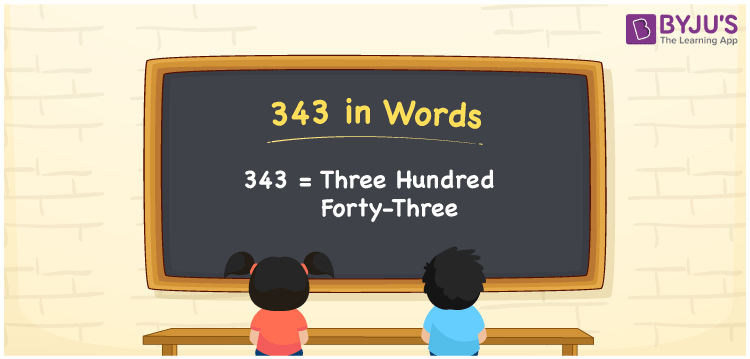# 343 in Words

343 in words is written as Three hundred forty-three. In both the International System of Numerals and the Indian System of Numerals, 343 is written as Three hundred forty-three. The number 343 is a Cardinal Number as it represents some quantity. For example, “there are 343 chairs in the room”.

 343 in Words Three hundred forty-three Three hundred forty-three in Number 343

## 343 in English Words

We write 343 in English Words using the letters of the English alphabet. Therefore, we read 343 in English as “Three hundred forty-three.”## How to Write 343 in Words?

To write 343 in words, we shall use the place value chart. In the place value chart, write 3 in the hundreds, 4 in the tens, and 3 in the ones, respectively. Now let us make a place value chart to write the number 343 in words.

 Hundreds Tens Ones 3 4 3

Thus, we can write the expanded form as

3 × Hundred + 4 × Ten + 3 × One

= 3 × 100 + 4 × 10 + 3 × 1

= 300 + 40 + 3

= 343

= Three hundred forty-three.

343 is a natural number, the successor of 342 and the predecessor of 344.

343 in words – Three hundred forty-three

• Is 343 an odd number? – Yes
• Is 343 an even number? – No
• Is 343 a perfect square number? – No
• Is 343 a perfect cube number? – No
• Is 343 a prime number? – No
• Is 343 a composite number? – Yes

## Frequently Asked Questions on 343 in Words

Q1

### How to write 343 in words?

343 in words is written as Three hundred forty-three.
Q2

### How to write 343 in the International and Indian System of Numerals?

In both, the system of numerals, 343 in words, is written as Three hundred forty-three.
Q3

### What is the preceding number of 343?

The number that precedes 343 is 342.